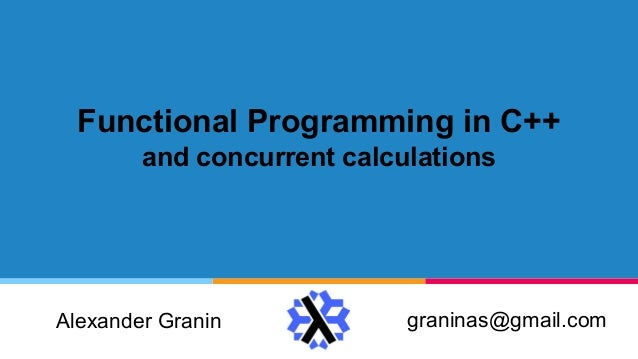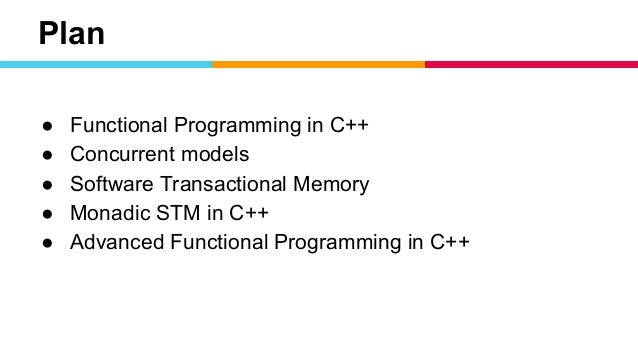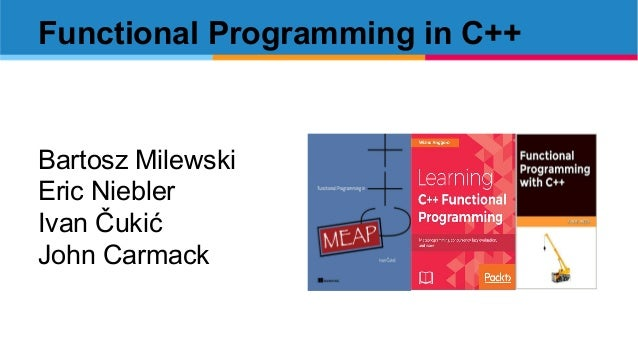Successfully reported this slideshow.Upcoming SlideShare
×

ofUpcoming SlideShare
Next

Share

# Functional programming in C++ LambdaNsk

See all

### Related Audiobooks

#### Free with a 30 day trial from Scribd

See all
• Be the first to like this

### Functional programming in C++ LambdaNsk

1. 1. Functional Programming in C++ and concurrent calculations graninas@gmail.comAlexander Granin
2. 2. Plan ● Functional Programming in C++ ● Concurrent models ● Software Transactional Memory ● Monadic STM in C++ ● Advanced Functional Programming in C++
3. 3. Functional Programming in C++ Bartosz Milewski Eric Niebler Ivan Čukić John Carmack
4. 4. template<template<typename> class Monad> auto run() { return DO(Monad, (x, unit(1)) (y, unit(2)) (z, DO(Monad, (x, unit(5)) (_, unit(x - 2)) )) (_, unit(x + y + z)) ); } Introduction to FP in C++: Monads! Evgeny Panasyuk https://github.com/evgeny-panasyuk/monad_do
5. 5. Concurrent models shared resource actor actor
6. 6. std::shared_ptr std::shared_ptrint refCounter;
7. 7. struct Chest { int iron; int copper; int coal; }; iron (1) copper (1) copper (1) iron (1) coal (1)
8. 8. struct Chest { std::atomic<int> iron; std::atomic<int> copper; std::atomic<int> coal; }; iron (1) copper (1) copper (1) iron (1) coal (1)
9. 9. struct Chest { std::atomic<int> iron; std::atomic<int> copper; std::atomic<int> coal; }; iron < 100 copper < 100 coal > 6 && iron > 3 coal > 6 && copper > 3 iron (1) copper (1) coal (3), copper (3) coal (3), iron (3) coal (1) coal < 100
10. 10. struct Chest { int iron; int copper; int coal; std::mutex lock; }; iron < 100 copper < 100 coal > 6 && iron > 3 coal > 6 && copper > 3 iron (1) copper (1) coal (3), copper (3) coal (3), iron (3) coal (1) coal < 100
11. 11. Software Transactional Memory Wyatt-STM https://github.com/bretthall/Wyatt-STM CppCon 2015: Brett Hall “Transactional Memory in Practice" cpp_stm_free https://github.com/graninas/cpp_stm_free C++ Russia 2018 (Spb): “Functional approach to STM”
12. 12. Transactions struct Chest { stm::TVar<int> iron; stm::TVar<int> copper; stm::TVar<int> coal; };
13. 13. struct Chest { stm::TVar<int> iron; stm::TVar<int> copper; stm::TVar<int> coal; }; STM-based concurrency
14. 14. stm::STML<stm::Unit> insert(stm::TVar<int> resource, int count); stm::STML<stm::Unit> extract( stm::TVar<int> resource1, int count1, stm::TVar<int> resource2, int count2 ); struct Chest { stm::TVar<int> iron; stm::TVar<int> copper; stm::TVar<int> coal; }; STM-based concurrency
15. 15. stm::STML<stm::Unit> insert(stm::TVar<int> resource, int count); stm::STML<stm::Unit> extract( stm::TVar<int> resource1, int count1, stm::TVar<int> resource2, int count2 ); stm::Context ctx; stm::atomically(ctx, insert(chest.iron, 1) ); // Thread 1 stm::atomically(ctx, insert(chest.copper, 1) ); // Thread 2 stm::atomically(ctx, insert(chest.coal, 1) ); // Thread 3 stm::atomically(ctx, extract(chest.coal, 3, chest.iron, 3) ); // Thread 4 stm::atomically(ctx, extract(chest.coal, 3, chest.copper, 3) ); // Thread 5 struct Chest { stm::TVar<int> iron; stm::TVar<int> copper; stm::TVar<int> coal; }; STM-based concurrency
16. 16. Monadic STM in C++
17. 17. template <typename A> STML<TVar<A>> newTVar(const A& val); STM: TVar operations newTVar :: a → STML (TVar a)
18. 18. template <typename A> STML<TVar<A>> newTVar(const A& val); template <typename A> STML<A> readTVar(const TVar<A>& tvar); STM: TVar operations newTVar :: a → STML (TVar a) readTVar :: TVar a → STML a
19. 19. template <typename A> STML<TVar<A>> newTVar(const A& val); template <typename A> STML<A> readTVar(const TVar<A>& tvar); template <typename A> STML<Unit> writeTVar(const TVar<A>& tvar, const A& val); STM: TVar operations newTVar :: a → STML (TVar a) readTVar :: TVar a → STML a writeTVar :: TVar a → a → STML ()
20. 20. STM: Monadically composable transactions transaction :: STML Int transaction = do tvar ← newTVar 10 readTVar tvar STML<int> transaction() { STML<TVar<int>> tvarTrans = newTVar(10); // return readTVar(tvarTrans); // ???? }
21. 21. STM: Monadically composable transactions transaction :: STML Int transaction = do tvar ← newTVar 10 readTVar tvar transaction :: STML Int transaction = bind (newTVar 10) (tvar → readTVar tvar) STML<int> transaction() { STML<TVar<int>> tvarTrans = newTVar(10); // return readTVar(tvarTrans); // ???? } STML<int> transaction() { STML<TVar<int>> tvarTrans = newTVar(10); return bind(tvarTrans, [](TVar<int> tvar) { return readTVar(tvar); }); }
22. 22. Sample: Extract resources transaction STML<Unit> extract (TVar<int> resource1, int count1, TVar<int> resource2, int count2) { STML<Unit> t1 = extractResource (resource1, count1); STML<Unit> t2 = extractResource (resource2, count2); return bind (t1, [=](Unit) { return t2; }); } STML<Unit> extractResource (TVar<int> resource, int count) { STML<int> trans1 = readTVar (resource); return bind (trans1, [=](int value) { return value >= count ? writeTVar (recource, value - count) : retry(); }); }
23. 23. STM: C++ Interface template <typename A, typename B> STML<B> bind(const STML<A> ma, const std::function<STML<B>(A)>& f); template <typename A> STML<A> pure(const A& val); template <typename A> STML<A> retry(); template <typename A> STML<TVar<A>> newTVar(const A& val); template <typename A> STML<A> readTVar(const TVar<A>& tvar); template <typename A> STML<Unit> writeTVar(const TVar<A>& tvar, const A& val);
24. 24. Advanced Functional Programming in C++
25. 25. Design Pattern: eDSL Language ScenarioComposition Environment 1 Environment 2 ... Interpreting (Evaluation)
26. 26. template <typename A, typename Ret> struct NewTVar { A val; std::function<Ret(TVar<A>)> next; }; template <typename A, typename Ret> struct ReadTVar { TVar<A> tvar; std::function<Ret(A)> next; }; template <typename A, typename Ret> struct WriteTVar { TVar<A> tvar; A val; std::function<Ret(fp::Unit)> next; }; template <typename A, typename Ret> struct Retry { }; STM eDSL
27. 27. template <class Ret> struct STMF { std::variant<NewTVar <std::any, Ret>, ReadTVar <std::any, Ret>, WriteTVar <std::any, Ret>, Retry <std::any, Ret> > stmf; }; STM eDSL Generalized ADT data STMF next where NewTVar :: a -> (TVar a -> next) -> STMF next WriteTVar :: TVar a -> a -> next -> STMF next ReadTVar :: TVar a -> (a -> next) -> STMF next Retry :: STMF next
28. 28. template <typename A, typename B> struct StmfFunctorVisitor { STMF<B> result; void operator() (const NewTVar<std::any, STML<A>>& method); void operator() (const ReadTVar<std::any, STML<A>>& method); void operator() (const WriteTVar<std::any, STML<A>>& method); void operator() (const Retry <std::any, STML<A>>&); }; template <typename A, typename B> STMF<B> fmap(const std::function<B(A)>& f, const STMF<A>& method) { StmfFunctorVisitor<A, B> visitor(f); std::visit(visitor, method.stmf); return visitor.result; } STM eDSL ADT interpreting (“Pattern Matching”)
29. 29. Monadic STML chains transaction :: STML Int transaction = do tvar ← newTVar 42 v1 ← readTVar tvar v2 ← pure 10 pure (v1 + v2) STML<int> transaction() { return bind (newTVar (42), [=](TVar<int> tvar) { return bind (readTVar (tvar), [=](int v1) { return bind (pure (10), [=](int v2) { return pure (v1 + v2); }); }); }); }
30. 30. STML<int> ↳ STML<TVar<int>> ↳ STML<int> ↳ STML<int> ↳ STML<int> Recursive STML type STML<int> transaction() { return bind (newTVar (42), [=](TVar<int> tvar) { return bind (readTVar (tvar), [=](int v1) { return bind (pure (10), [=](int v2) { return pure (v1 + v2); }); }); }); }
31. 31. STML<int> [](){} ↳ STML<TVar<int>> [](){} ↳ STML<int> [](){} ↳ STML<int> [](){} ↳ STML<int> Recursive STML type (lambda-enclosed) STML<int> transaction() { return bind (newTVar (42), [=](TVar<int> tvar) { return bind (readTVar (tvar), [=](int v1) { return bind (pure (10), [=](int v2) { return pure (v1 + v2); }); }); }); }
34. 34. Free monads -- Free monad, normal form data Free f a = Pure a | Bind (f (Free f a)) Binding complexity: O(n2 ) <stm/free/stm.h>
35. 35. Free monads -- Free monad, normal form data Free f a = Pure a | Bind (f (Free f a)) -- Free monad, Church-encoded form data ChurchFree f a = ChurchFree { runChurch :: forall z. (a -> z) -> (f z -> z) -> z } Binding complexity: O(n2 ) <stm/free/stm.h> Binding complexity: O(n) <stm/church/stm.h> (10x faster)
36. 36. Free monads -- Free monad, normal form data Free f a = Pure a | Bind (f (Free f a)) -- Free monad, Church-encoded form data ChurchFree f a = ChurchFree { runChurch :: forall z. (a -> z) -> (f z -> z) -> z } -- Free monad, Scott-encoded form data Free a = Free { runFree :: forall u . (a -> u) -> (f (Free f a) -> u) -> u } Binding complexity: O(n2 ) <stm/free/stm.h> Binding complexity: O(n) <stm/church/stm.h> (10x faster) Binding complexity: O(n2 )
37. 37. STML Free monad type (normal form) -- Free monad, normal form data Free f a = Pure a | Bind (f (Free f a)) -- STML Free monad type type STML a = Free STMF a
38. 38. STML Free monad type (normal form) -- Free monad, normal form data Free f a = Pure a | Bind (f (Free f a)) -- STML Free monad type type STML a = Free STMF a f ~ STMF f (Free f a) ~ STMF (Free STMF a) f (Free f a) ~ STMF (STML a)
39. 39. STML Free monad type (normal form) -- Free monad, normal form data Free f a = Pure a | Bind (f (Free f a)) -- STML Free monad type type STML a = Free STMF a f ~ STMF f (Free f a) ~ STMF (Free STMF a) f (Free f a) ~ STMF (STML a) template <typename Ret> struct STML { std::variant<PureF<Ret>, BindF<Ret>> stml; }; template <typename Ret> struct PureF { Ret ret; }; template <typename Ret> struct BindF { STMF<STML<Ret>> stmf; };
40. 40. STML Free monad type (normal form) template <typename Ret> struct STML { std::variant<PureF<Ret>, BindF<Ret>> stml; }; template <typename Ret> struct PureF { Ret ret; }; template <typename Ret> struct BindF { STMF<STML<Ret>> stmf; }; template <class Ret> struct STMF { std::variant<NewTVar <std::any, Ret>, ReadTVar <std::any, Ret>, WriteTVar <std::any, Ret>, Retry <std::any, Ret> > stmf; }; template <typename A, typename Ret> struct NewTVar { A val; std::function<Ret(TVar<A>)> next; };
41. 41. STML monadic binding template <typename A, typename B> struct BindStmlVisitor; template <typename A, typename B> struct BindStmfVisitor; template <typename A, typename B> STML<B> bind(const STML<A>& stml, const std::function<STML<B>(A)>& f) { BindStmlVisitor<A, B> visitor(f); std::visit(visitor, stml.stml); return visitor.result; }
42. 42. Thank you for watching! Alexander Granin graninas@gmail.com

Total views

447

On Slideshare

0

From embeds

0

Number of embeds

18

2

Shares

0